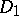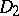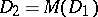# Decomposition

(diff) ← Older revision | Latest revision (diff) | Newer revision → (diff)
In discrete geometry one frequently considers the decomposition of some space into closed domains which cover the entire space and whose pairwise intersections have no interior points (they may have common boundary points). For example, if one fixes any point lattice in the Euclidean spaceand assigns to each point of the lattice those points of the space that are less distant from this point than from any other point of the lattice, then the so-called Dirichlet–Voronoi decomposition is obtained. A decomposition of a spaceis called regular if for any domainsandin it there is a motionfor whichand. See also Voronoi lattice types.
In combinatorial geometry there are several problems and results relating to particular decompositions of certain sets. An example of such a problem is the Borsuk problem: Is it possible to divide any set of diameterinintoparts, each of them having diameter less than? Inthere are bounded sets for which a decomposition into a smaller number of such parts is impossible. Any decomposition determines a certain covering (cf. Covering (of a set)) and from any covering one can obtain a decomposition. Decompositions are closely connected with the illumination problem and the Hadwiger hypothesis.